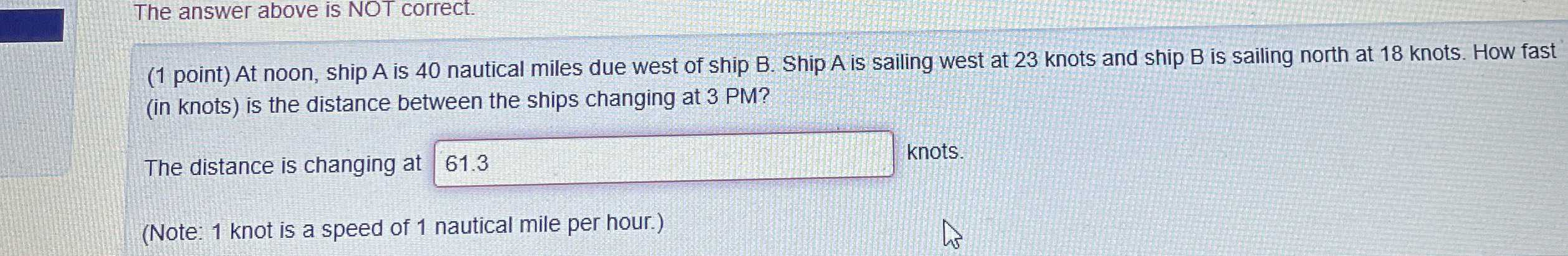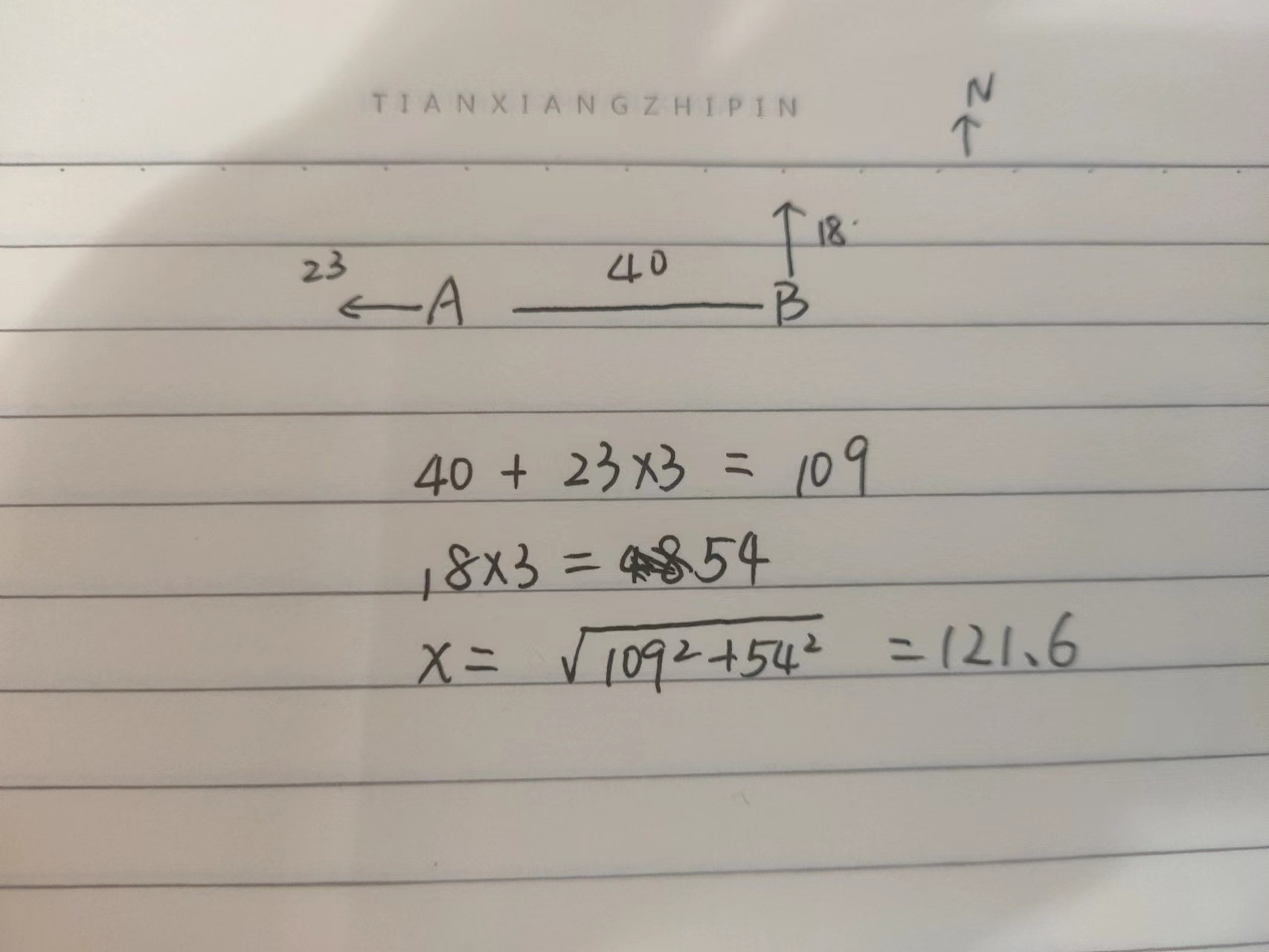### Still have math questions?

Trigonometry
QuestionThe answer above is NOT correct. (1 point) At noon, ship A is $$40$$ nautical miles due west of ship B. Ship A is sailing west at $$23$$ knots and ship B is sailing north at $$18$$ knots. How fast (in knots) is the distance between the ships changing at $$3$$ PM?

The distance is changing at _______

(Note: $$1$$ knot is a speed of $$1$$ nautical mile per hour.)